## General Question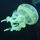# If somebody choose two digit and three digit numbers and their difference is 989.What will be their sum? a) 1000 b)1010 c) 1006?

Asked by Daniyal963 (9) June 15th, 2019

If somebody choose two digit and three digit numbers and their difference is 989.What will be their sum?
a) 1000
b)1010
c) 1006

Observing members: 0Composing members: 0None of the above.

Let x be the three digit number and y be the two digit number.
x – y =989. x = 989 + y. x is obviously greater than 989 and less than 1000 (since it is three digits). Since y is a two digit number, y is at least 10 and x is at least 989 + 10 = 999. We can’t go any higher, so x =999 and y=10. x+y = 999+10 = 1009

LostInParadise (27369)“Great Answer” (7) Flag as…A quick way to see that the choices are not correct is to realize that since the difference between the numbers, 989, is an odd number, the sum must also be an odd number. All the choices are even numbers.

LostInParadise (27369)“Great Answer” (8) Flag as…LostlnParadise I’ve tried all these approaches before…. I wasn’t able to solve it cuz it’s a bit tricky! Although this question and all the options are valid ! The answer to this question lies in this question ! And the way I know it’s correct is because the question came in my exam,so the question never comes wrong, it’s checked several times !

Daniyal963 (9)“Great Answer” (1) Flag as…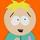@LostInParadise is correct, and I am impressed with the elegance of his second post.

filmfann (47289)“Great Answer” (0) Flag as…@Daniyal963 , I don’t know what else to say. You have to agree that 999 and 10 is a solution, and, if you can follow my logic, you will see there is no other solution. Have you studied algebra? Without algebra this is a bit of tricky question.

LostInParadise (27369)“Great Answer” (3) Flag as…Is 10.5 a two digit number?
If you think so, then 999.5 is your other number, and the sum is (b) 1010, but it’s really not a correct solution.

filmfann (47289)“Great Answer” (0) Flag as…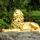No, 10.5 is not a 2-digit number.

Zaku (24383)“Great Answer” (1) Flag as…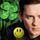@LostInParadise Your even-odd approach is beautiful! If the difference is odd then one number must be odd, the other even. Therefore the sum must be odd.
All the choices given are even so none are correct.

LuckyGuy (38050)“Great Answer” (1) Flag as…Is it possible one of the choices was supposed to be 1009?

LuckyGuy (38050)“Great Answer” (0) Flag as…Here is another way of thinking about the problem. If I were really smart, I would have thought of it immediately. If there were no three digit and two digit limitations, there would be infinitely many solutions for numbers whose difference is 989. For there to be a unique solution, we must be at a boundary.The largest difference we can get is from the largest three digit number and the smallest two digit number, 999 and 10. A trivial twist on the problem is to ask for a three digit number and two digit number whose difference is 1. The smallest difference is between the smallest three digit number 100 and the largest two digit number, 99.

LostInParadise (27369)“Great Answer” (1) Flag as…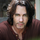@Daniyal963 I think there was a typo in either your test or your transcription because every version of this question I was able to find elsewhere includes 1009 as a possible answer.

JeSuisRickSpringfield (6535)“Great Answer” (0) Flag as…or